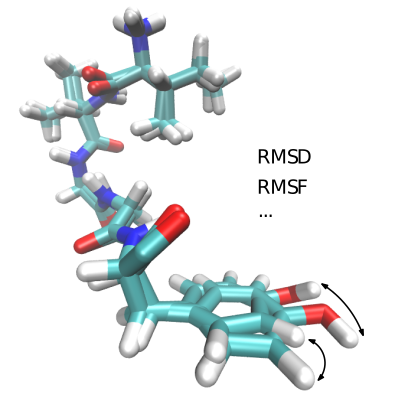Structural Bioinformatics Library Template C++ / Python API for developping structural bioinformatics applications.
Molecular_distances

Algorithms computing different kind of distances between molecular conformations. Reference ManualUser Manual . Authors: F. Cazals and T. DreyfusMain classes:

• T_Binet_Cauchy_kernel_score : Model of Distances for defining distance between two conformations
• T_External_distance : Distance computed from an external executable (sbl-conf-distance.exe)
• T_Get_particle_default : Model of Distance for defining distance between two conformations
• T_Least_RMSD_cartesian : Model of Distances for defining distance between two conformations
• T_Least_RMSD_cartesian_with_chirality : Model of Distances for defining distance between two conformations
• Molecular_alignment_default : Make an alignment between two molecules with the same numbers of atoms, with the same order.
• T_RMSD_comb_edge_weighted : Given a set of motifs
• T_Squared_angular_internal_distance : Model of distance between angles of two conformations given with their internal coordinates
• T_Angular_internal_distance : Model of Distance between angles of two conformations given with their internal coordinates
• T_Squared_RMSD_internal_distance : Model of Distance for defining distance between two conformations
• T_Squared_RMSD_cartesian_3 : Squared RMSD distance between two 3D point clouds
• T_RMSD_cartesian_3 : RMSD distance between two 3D point clouds
• T_Squared_Least_RMSD_cartesian_3 : Perform a 3D rigid registration between two 3D point clouds, then computes the squared RMSD distance between the two aligned clouds
• T_Least_RMSD_cartesian_3 : Perform a 3D rigid registration between two 3D point clouds, then computes the RMSD distance between the two aligned clouds